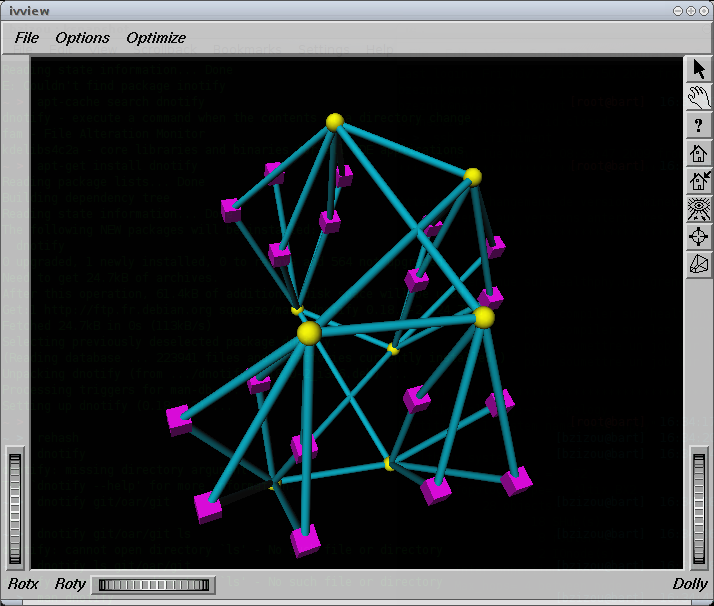# Use cases

## OpenMPI + affinity

We saw that the Linux kernel seems to be incapable of using correctly all the CPUs from the cpusets.

Indeed, reserving 2 out of 8 cores on a node and running a code that uses 2 process, these 2 process where not well assigned to each cpu. We had to give the CPU MAP to OpenMPI to do cpu_affinity:

``` i=0 ; oarprint core -P host,cpuset -F "% slot=%" | while read line ; do echo "rank \$i=\$line"; ((i++)); done > affinity.txt

[user@node12 tmp]\$ mpirun -np 8 --mca btl openib,self -v -display-allocation -display-map  --machinefile \$OAR_NODEFILE -rf affinity.txt /home/user/espresso-4.0.4/PW/pw.x < BeO_100.inp```

## NUMA topology optimization

In this use case, we've got a numa host (an Altix 450) having a “squared” topology: nodes are interconnected by routers like in this view:In yellow, “routers”, in magenta, “nodes” (2 dual-core processors per node)

Routers interconnect IRUS (chassis) on which the nodes are plugged (4 or 5 nodes per IRU).

What we want is that for jobs that can enter into 2 IRUS or less, minimize the distance between the resources (ie use IRUS that have only one router interconnexion between them). The topology may be siplified as follows:The idea is to use moldable jobs and an admission rule that converts automatically the user requests to a moldable job. This job uses 2 resource properties: numa_x and numa_y that may be analogue to the square coordinates. What we want in fact, is the job that ends the soonest between a job running on an X or on a Y coordinate (we only want vertical or horizontal placed jobs).

The numa_x and numa_y properties are set up this way (pnode is a property corresponding to physical nodes):

{|border=“1” !pnode !iru !numa_x !numa_y

For example, the following requested ressources:

` -l /core=16`

will result into:

` -l /numa_x=1/pnode=4/cpu=2/core=2 -l /numa_y=1/pnode=4/cpu=2/core=2`

Here is the admission rule making that optimization:

``` # Title : Numa optimization
# Description : Creates a moldable job to take into account the "squared" topology of an Altix 450
my \$n_core_per_cpus=2;
my \$n_cpu_per_pnode=2;
if (grep(/^itanium\$/, @{\$type_list}) && (grep(/^manual\$/, @{\$type_list}) == "") && \$#\$ref_resource_list == 0){
print "[ADMINSSION RULE] Optimizing for numa architecture (use \\"-t manual\\" to disable)";
my \$resources_def=\$ref_resource_list->;
my \$core=0;
my \$cpu=0;
my \$pnode=0;
foreach my \$r (@{\$resources_def->}) {
foreach my \$resource (@{\$r->{resources}}) {
if (\$resource->{resource} eq "core") {\$core=\$resource->{value};}
if (\$resource->{resource} eq "cpu") {\$cpu=\$resource->{value};}
if (\$resource->{resource} eq "pnode") {\$pnode=\$resource->{value};}
}
}
# Now, calculate the number of total cores
my \$n_cores=0;
if (\$pnode == 0 && \$cpu != 0 && \$core == 0) {
\$n_cores = \$cpu*\$n_core_per_cpus;
}
elsif (\$pnode != 0 && \$cpu == 0 && \$core == 0) {
\$n_cores = \$pnode*\$n_cpu_per_pnode*\$n_core_per_cpus;
}
elsif (\$pnode != 0 && \$cpu == 0 && \$core != 0) {
\$n_cores = \$pnode*\$core;
}
elsif (\$pnode == 0 && \$cpu != 0 && \$core != 0) {
\$n_cores = \$cpu*\$core;
}
elsif (\$pnode == 0 && \$cpu == 0 && \$core != 0) {
\$n_cores = \$core;
}
else { \$n_cores = \$pnode*\$cpu*\$core; }
print "[ADMINSSION RULE] You requested \$n_cores cores\";
if (\$n_cores > 32) {
print "[ADMISSION RULE] Big job (>32 cores), no optimization is possible";
}else{
print "[ADMISSION RULE] Optimization produces: /numa_x=1/\$pnode/\$cpu/\$core
/numa_y=1/\$pnode/\$cpu/\$core";

my @newarray=eval(Dumper(@{\$ref_resource_list}->));
push (@{\$ref_resource_list},@newarray);
unshift(@{%{@{@{@{\$ref_resource_list}->}->}->}->{resources}},{'resource' => 'numa_x','value' => '1'});
unshift(@{%{@{@{@{\$ref_resource_list}->}->}->}->{resources}},{'resource' => 'numa_y','value' => '1'});
}
}```

# Users tips

## oarsh completion

Tip based on an idea from Jerome Reybert

In order to complete nodes names in a oarsh command, add these lines in your .bashrc

```function _oarsh_complete_() {
if [ -n "\$OAR_NODEFILE" -a "\$COMP_CWORD" -eq 1 ]; then
local word=\${comp_words[comp_cword]}
local list=\$(cat \$OAR_NODEFILE | uniq | tr '\n' ' ')
fi
}
complete -o default -F _oarsh_complete_ oarsh```

Then try oarsh <TAB>

## OAR aware shell prompt for Interactive jobs

If you want to have a bash prompt with your job id and the remaining walltime then you can add in your ~/.bashrc:

```if [ "\$PS1" ]; then
__oar_ps1_remaining_time(){
if [ -n "\$OAR_JOB_WALLTIME_SECONDS" -a -n "\$OAR_NODE_FILE" -a -r "\$OAR_NODE_FILE" ]; then
DATE_NOW=\$(date +%s)
DATE_JOB_START=\$(stat -c %Y \$OAR_NODE_FILE)
DATE_TMP=\$OAR_JOB_WALLTIME_SECONDS
((DATE_TMP = (DATE_TMP - DATE_NOW + DATE_JOB_START) / 60))
echo -n "\$DATE_TMP"
fi
}
PS1='[\u@\h|\W]\$([ -n "\$OAR_NODE_FILE" ] && echo -n "(\[\e[1;32m\]\$OAR_JOB_ID\[\e[0m\]-->\[\e[1;34m\]\$(__oar_ps1_remaining_time)mn\[\e[0m\])")\\$ '
if [ -n "\$OAR_NODE_FILE" ]; then
echo "[OAR] OAR_JOB_ID=\$OAR_JOB_ID"
sort \$OAR_NODE_FILE | uniq -c | awk '{printf("      %s*%d", \$2, \$1)}END{printf("\n")}' | sed -e 's/,\$//'
fi
fi```

Then the prompt inside an Interactive job will be like:

`  [capitn@node006~](3101-->29mn)\$`

## Many small jobs grouping

Many small jobs of a few seconds may be painful for the OAR system. OAR may spend more time scheduling, allocating and launching than the actual computation time for each job.

Gabriel Moreau developed a script that may be useful when you have a large set of small jobs. It groups you jobs into a unique bigger OAR job:

For a more generic approach you can use Cigri, a grid middleware running onto OAR cluster(s) that is able to automatically group parametric jobs. Cigri is currently in a re-writing process and a new public release is planned for the end of 2012.

## Overcoming quoting issues n oarsub commands

One may get a bit of a weird behavior when trying to submit a series of jobs through a bash script. For instance:

```#!/bin/bash

for d in ../test/*/ ; do
CMD="oarsub -p \"cputype='xeon'\" -l /nodes=1,walltime=04:00:00 -S \"./myscript.sh \$d/in \$d/out\""
echo \$CMD
\$CMD
done```

This does not work, due to quoting issues.

A solution is to use a bash arrays to define the command, as follows:

```#!/bin/bash

for d in ../test/*/ ; do
declare -a CMD
CMD=(oarsub -p "cputype='xeon'" -l /nodes=1,walltime=04:00:00 -S "./myscript.sh \$d/in \$d/out")
"\${CMD[@]}"
done```

This works.

## Environment variables through oarsh